Courses

# Shear and Moment Equations and Diagrams Mechanical Engineering Notes | EduRev

## Mechanical Engineering : Shear and Moment Equations and Diagrams Mechanical Engineering Notes | EduRev

The document Shear and Moment Equations and Diagrams Mechanical Engineering Notes | EduRev is a part of the Mechanical Engineering Course Engineering Mechanics - Notes, Videos, MCQs & PPTs.
All you need of Mechanical Engineering at this link: Mechanical Engineering

Shear Force and Bending Moment Diagrams

You probably can tell from the examples previously that the shear force SF and bending moment BMvaries along the beam, due to the varying loads.

From an engineer’s point of view, you would want to find out where the maximum SF or BM is – i.e. the weakest part of the beam. This is so that you can design to ensure that it’s safe!

Likewise you would want to know where the minimum SF or BM is, so that you will not overdesign that portion of the beam

So how do we conveniently see the SF and BM along the beam? Well that’s what the SF and BMdiagrams are for!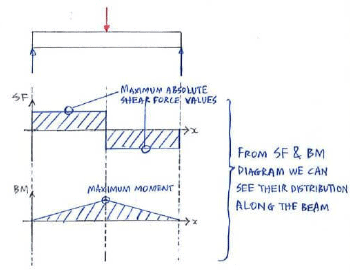There are 2 methods to construct the SF and BM diagrams:

Method 1: Equation approach

In this method, you basically obtain the expression for SF (or V) and BM (or M) as a function of the distance x from the left end of the beam. The equations are obtained using the equations of equilibrium such that the internal forces ensure equilibrium of the section cut: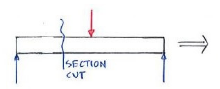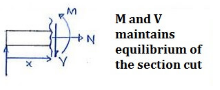The equations obtained are then used to construct the SF and BM diagrams. Note that you will need one equation for every single change in loading (i.e. when a new force comes in, as you move from the left to right of the beam):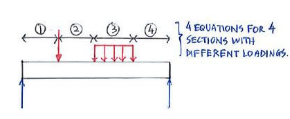It might seem vague at the moment, but it will make more sense once you work through an example.

Method 2: Direct method

This is the recommended method but it really takes practice to master. Basically this method works by directly constructing the SF diagram using the FBD, and BM diagram using both the SF diagram and FBD.

Two key relationships for this method are as follows:

dV/dx = -w  dM/dx = v

The relationships basically say that the gradient of the SF diagram is equal to the –ve of the distributed load, while the gradient of the BM diagram is equal to the SF: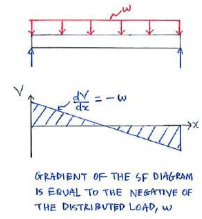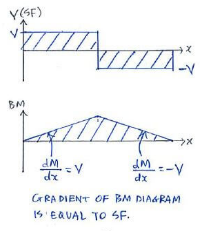We also present to you a few examples of how different forces acting on a beam are represented in SFand BM diagrams: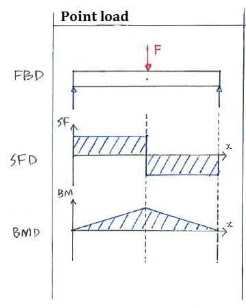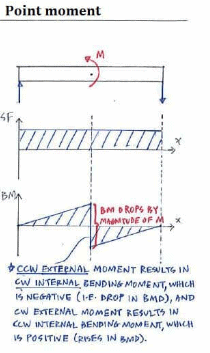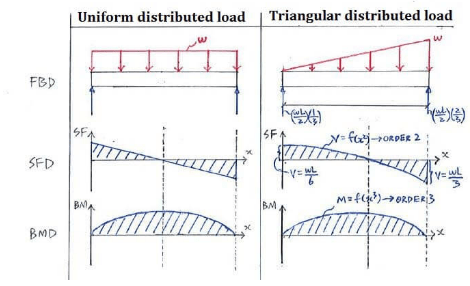So how do we actually construct the SF and BM diagrams directly?

Offer running on EduRev: Apply code STAYHOME200 to get INR 200 off on our premium plan EduRev Infinity!

,

,

,

,

,

,

,

,

,

,

,

,

,

,

,

,

,

,

,

,

,

;